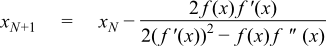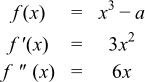#Boost C++ Libraries

...one of the most highly regarded and expertly designed C++ library projects in the world.

This is the documentation for an old version of boost. Click here for the latest Boost documentation.

#### Root Finding With Derivatives

##### Synopsis

```#include <boost/math/tools/roots.hpp>
```

```namespace boost{ namespace math{ namespace tools{

template <class F, class T>
T newton_raphson_iterate(F f, T guess, T min, T max, int digits);

template <class F, class T>
T newton_raphson_iterate(F f, T guess, T min, T max, int digits, boost::uintmax_t& max_iter);

template <class F, class T>
T halley_iterate(F f, T guess, T min, T max, int digits);

template <class F, class T>
T halley_iterate(F f, T guess, T min, T max, int digits, boost::uintmax_t& max_iter);

template <class F, class T>
T schroeder_iterate(F f, T guess, T min, T max, int digits);

template <class F, class T>
T schroeder_iterate(F f, T guess, T min, T max, int digits, boost::uintmax_t& max_iter);

}}} // namespaces
```
##### Description

These functions all perform iterative root finding: `newton_raphson_iterate` performs second order Newton Raphson iteration, while `halley_iterate` and `schroeder_iterate` perform third order Halley and Schroeder iteration respectively.

The functions all take the same parameters:

Parameters of the root finding functions

F f

Type F must be a callable function object that accepts one parameter and returns a boost::math::tuple:

For the second order iterative methods (Newton Raphson) the boost::math::tuple should have two elements containing the evaluation of the function and it's first derivative.

For the third order methods (Halley and Schroeder) the boost::math::tuple should have three elements containing the evaluation of the function and its first and second derivatives.

T guess

The initial starting value.

T min

The minimum possible value for the result, this is used as an initial lower bracket.

T max

The maximum possible value for the result, this is used as an initial upper bracket.

int digits

The desired number of binary digits.

uintmax_t max_iter

An optional maximum number of iterations to perform.

When using these functions you should note that:

• They may be very sensitive to the initial guess, typically they converge very rapidly if the initial guess has two or three decimal digits correct. However convergence can be no better than bisection, or in some rare cases even worse than bisection if the initial guess is a long way from the correct value and the derivatives are close to zero.
• These functions include special cases to handle zero first (and second where appropriate) derivatives, and fall back to bisection in this case. However, it is helpful if F is defined to return an arbitrarily small value of the correct sign rather than zero.
• If the derivative at the current best guess for the result is infinite (or very close to being infinite) then these functions may terminate prematurely. A large first derivative leads to a very small next step, triggering the termination condition. Derivative based iteration may not be appropriate in such cases.
• These functions fall back to bisection if the next computed step would take the next value out of bounds. The bounds are updated after each step to ensure this leads to convergence. However, a good initial guess backed up by asymptotically-tight bounds will improve performance no end rather than relying on bisection.
• The value of digits is crucial to good performance of these functions, if it is set too high then at best you will get one extra (unnecessary) iteration, and at worst the last few steps will proceed by bisection. Remember that the returned value can never be more accurate than f(x) can be evaluated, and that if f(x) suffers from cancellation errors as it tends to zero then the computed steps will be effectively random. The value of digits should be set so that iteration terminates before this point: remember that for second and third order methods the number of correct digits in the result is increasing quite substantially with each iteration, digits should be set by experiment so that the final iteration just takes the next value into the zone where f(x) becomes inaccurate.
• Finally: you may well be able to do better than these functions by hand-coding the heuristics used so that they are tailored to a specific function. You may also be able to compute the ratio of derivatives used by these methods more efficiently than computing the derivatives themselves. As ever, algebraic simplification can be a big win.

##### Newton Raphson Method

Given an initial guess x0 the subsequent values are computed using:Out of bounds steps revert to bisection of the current bounds.

Under ideal conditions, the number of correct digits doubles with each iteration.

##### Halley's Method

Given an initial guess x0 the subsequent values are computed using:Over-compensation by the second derivative (one which would proceed in the wrong direction) causes the method to revert to a Newton-Raphson step.

Out of bounds steps revert to bisection of the current bounds.

Under ideal conditions, the number of correct digits trebles with each iteration.

##### Schroeder's Method

Given an initial guess x0 the subsequent values are computed using:Over-compensation by the second derivative (one which would proceed in the wrong direction) causes the method to revert to a Newton-Raphson step. Likewise a Newton step is used whenever that Newton step would change the next value by more than 10%.

Out of bounds steps revert to bisection of the current bounds.

Under ideal conditions, the number of correct digits trebles with each iteration.

##### Example

Lets suppose we want to find the cube root of a number, the equation we want to solve along with its derivatives are:To begin with lets solve the problem using Newton Raphson iterations, we'll begin be defining a function object that returns the evaluation of the function to solve, along with its first derivative:

```template <class T>
struct cbrt_functor
{
cbrt_functor(T const& target) : a(target){}
boost::math::tuple<T, T> operator()(T const& z)
{
T sqr = z * z;
return std::tr1::make_tuple(sqr * z - a, 3 * sqr);
}
private:
T a;
};
```

Implementing the cube root is fairly trivial now, the hardest part is finding a good approximation to begin with: in this case we'll just divide the exponent by three:

```template <class T>
T cbrt(T z)
{
using namespace std;
int exp;
frexp(z, &exp);
T min = ldexp(0.5, exp/3);
T max = ldexp(2.0, exp/3);
T guess = ldexp(1.0, exp/3);
int digits = std::numeric_limits<T>::digits;
}
```

Using the test data in libs/math/test/cbrt_test.cpp this found the cube root exact to the last digit in every case, and in no more than 6 iterations at double precision. However, you will note that a high precision was used in this example, exactly what was warned against earlier on in these docs! In this particular case its possible to compute f(x) exactly and without undue cancellation error, so a high limit is not too much of an issue. However, reducing the limit to ```std::numeric_limits<T>::digits * 2 / 3``` gave full precision in all but one of the test cases (and that one was out by just one bit). The maximum number of iterations remained 6, but in most cases was reduced by one.

Note also that the above code omits error handling, and does not handle negative values of z correctly. That will be left as an exercise for the reader!

Now lets adapt the functor slightly to return the second derivative as well:

```template <class T>
struct cbrt_functor
{
cbrt_functor(T const& target) : a(target){}
boost::math::tuple<T, T, T> operator()(T const& z)
{
T sqr = z * z;
return std::tr1::make_tuple(sqr * z - a, 3 * sqr, 6 * z);
}
private:
T a;
};
```

And then adapt the `cbrt` function to use Halley iterations:

```template <class T>
T cbrt(T z)
{
using namespace std;
int exp;
frexp(z, &exp);
T min = ldexp(0.5, exp/3);
T max = ldexp(2.0, exp/3);
T guess = ldexp(1.0, exp/3);
int digits = std::numeric_limits<T>::digits / 2;
Just to complete the picture, we could have called `schroeder_iterate` in the last example: and in fact it makes no difference to the accuracy or number of iterations in this particular case. However, the relative performance of these two methods may vary depending upon the nature of f(x), and the accuracy to which the initial guess can be computed. There appear to be no generalisations that can be made except "try them and see".